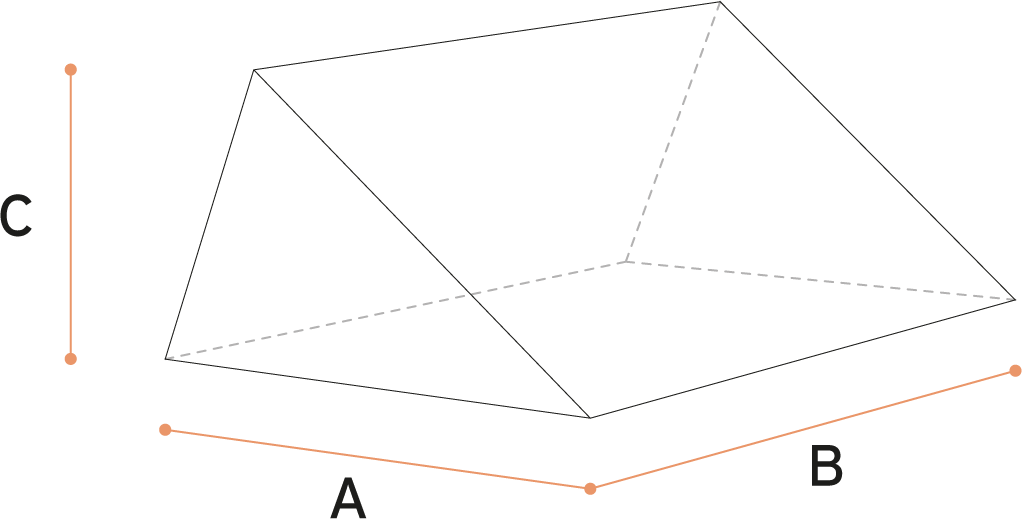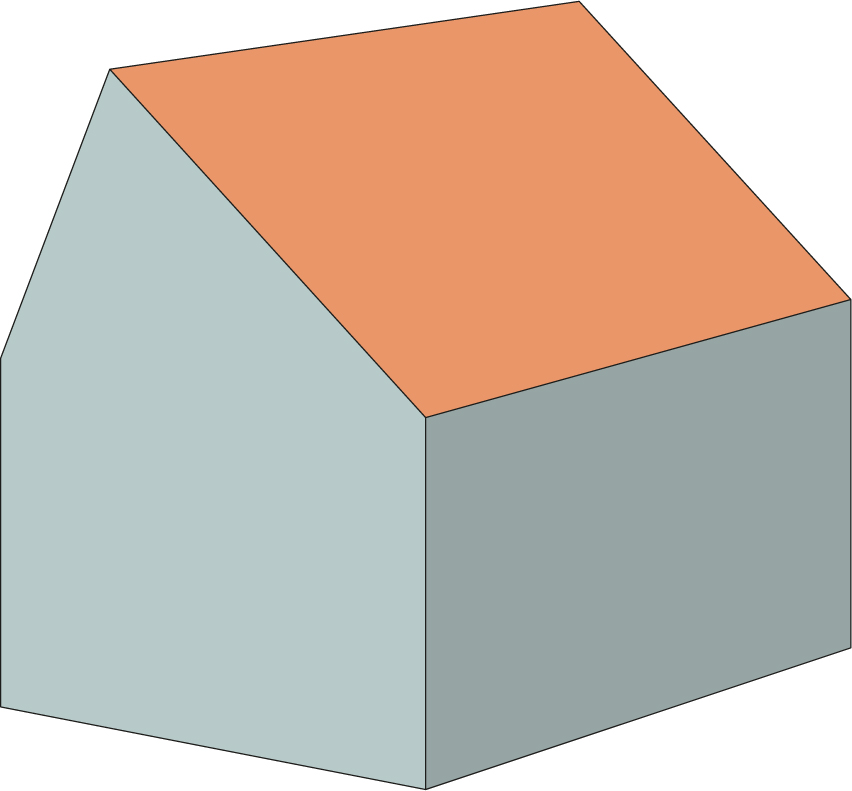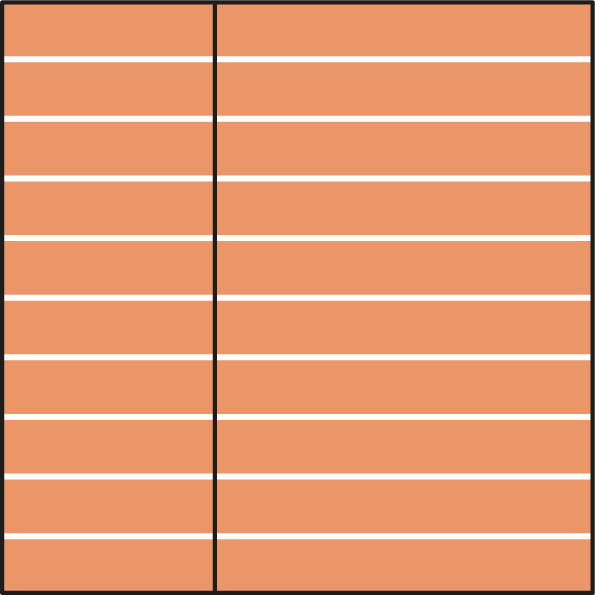# Asymmetrical gable roof volume calculator

## Asymmetrical gable roof | skeleton## Asymmetrical gable roof | formulaThe formula is also suitable for cubic feet.

## Calculate the volume of a Asymmetrical gable roof

Metic
– Metric form gives cubic meters / m3
– Fill out meters with decimals (e.g. 1 or 1.2 or 1.23)
– Decimals with a point ‘.’ , not a comma ‘,’

Imperial
– Imperial form gives cubic feet
– Fill out ‘0 inches’ if you have only feet.

## Asymmetrical gable roof | isometric view## Asymmetrical gable roof | top view## About the Asymmetrical gable roof

The asymmetrical gable roof is a variant of the regular gable roof. It’s similar to a gable roof in that it also consists of two sloping roof planes that intersect along the ridge to form an inverted ‘V’ (or Λ-shape).

The difference, however, is that its two roof planes aren’t the same. Its roof planes may vary in length and/or pitch (slope). In contrast, a symmetrical gable roof’s roof planes are equal in both length and pitch (slope).

A gable is the triangular portion of a facade above eave level between intersecting roof planes.

## Synonyms asymmetrical gable roof

You’ll hear different terms used for the asymmetrical gable roof, for example, catslide roof and saltbox roof.